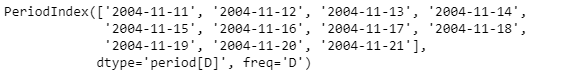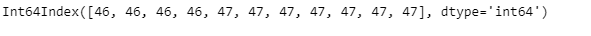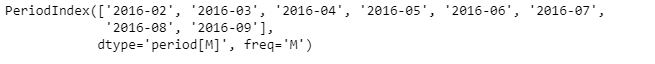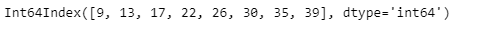# Python | Pandas PeriodIndex.week

• Last Updated : 06 Jan, 2019

Python is a great language for doing data analysis, primarily because of the fantastic ecosystem of data-centric python packages. Pandas is one of those packages and makes importing and analyzing data much easier.

Pandas` PeriodIndex.week` attribute return an Index object containing the ordinal value of week of each period element for the given PeriodIndex object.

Attention geek! Strengthen your foundations with the Python Programming Foundation Course and learn the basics.

To begin with, your interview preparations Enhance your Data Structures concepts with the Python DS Course. And to begin with your Machine Learning Journey, join the Machine Learning - Basic Level Course

Syntax : PeriodIndex.week

Parameters : None

Return : Index object

Example #1: Use `PeriodIndex.week` attribute to find out the ordinal value of week of each period element contained in the given PeriodIndex object.

 `# importing pandas as pd``import` `pandas as pd`` ` `# Create the PeriodIndex object``pidx ``=` `pd.PeriodIndex(start ``=``'2004-11-11 02:45:21 '``, ``               ``end ``=``'2004-11-21 8:45:29'``, freq ``=``'D'``)`` ` `# Print the PeriodIndex object``print``(pidx)`

Output :Now we will use the `PeriodIndex.week` attribute to find out the week’s ordinal value in the year for the given PeriodIndex object.

 `# return the week's ordinal value``pidx.week`

Output :As we can see in the output, the `PeriodIndex.week` attribute has returned an Index object containing the ordinal value of the week for each period element contained in the given PeriodIndex object.

Example #2: Use `PeriodIndex.week` attribute to find out the ordinal value of week of each period element contained in the given PeriodIndex object.

 `# importing pandas as pd``import` `pandas as pd`` ` `# Create the PeriodIndex object``pidx ``=` `pd.PeriodIndex(start ``=``'2016-2-12 11:12:02'``, ``           ``end ``=``'2016-09-12 11:32:12'``, freq ``=``'M'``)`` ` `# Print the PeriodIndex object``print``(pidx)`

Output :Now we will use the `PeriodIndex.week` attribute to find out the week’s ordinal value in the year for the given PeriodIndex object.

 `# return the week's ordinal value``pidx.week`

Output :As we can see in the output, the `PeriodIndex.week` attribute has returned an Index object containing the ordinal value of the week for each period element contained in the given PeriodIndex object.

My Personal Notes arrow_drop_up